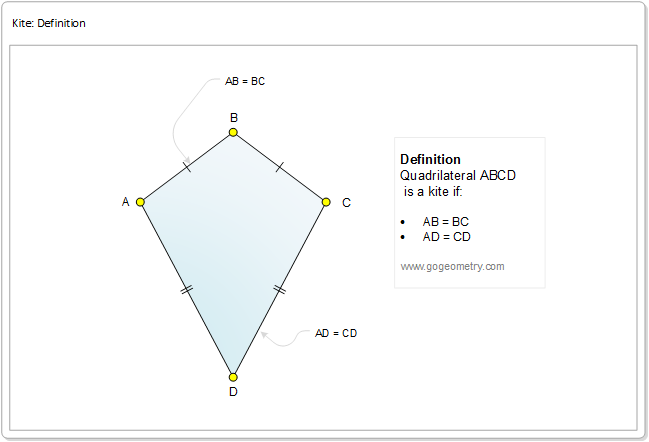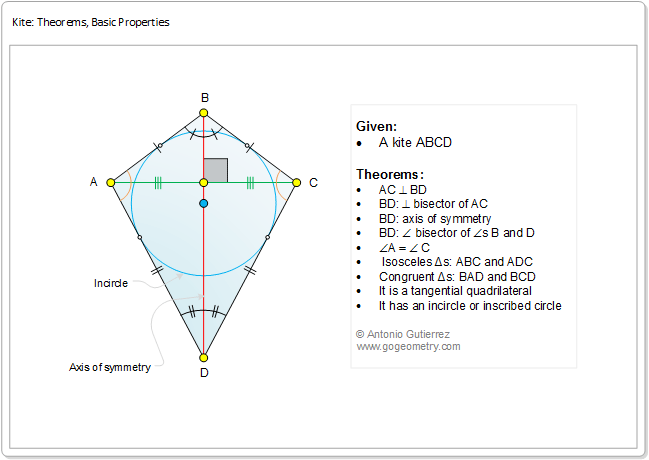A kite is a quadrilateral whose four sides can be grouped into two pairs of equal adjacent sides.Kite: Basic Theorems and Properties

Triangle, Isosceles, Midpoint, Congruence, Symmetry, Diagonal, Angle, Angle bisector, Perpendicular, Perpendicular bisector, Circle, Incircle, Inscribed circle, Tangent line, Tangential quadrilateral, Tangency point.